Hide

Week 10 - Conversions and Hidden Words

Welcome to week 10. Specially selected for the September 2018 11+ in Bucks, this week, we look at ALL NEW Hidden Words, mostly new Anagrams, and not new Verbs; we also continue with non-Verbal Reasoning; and we look at converting between units of volume, weight and length. There is also a link to the Book Trust’s top 100 books for children.

VolumeSometimes we need to measure liquids in large amounts called litres, (L) but sometimes we need to measure them in smaller amounts called millilitres (ml).

For example, you don’t want to have to carry a 1L bottle of water with you to school every day, so you carry it in bottles that contain less than 1L of water.

So you can have:

A portable water bottle that contain 250 ml of water.

Take medicine in 5 ml spoonfuls.To convert bigger unit into smaller units, multiply.

To convert 1 litre into millitres, multiply by 1,000.

1L x 1,000 = 1,000ml. They remain equal, but different.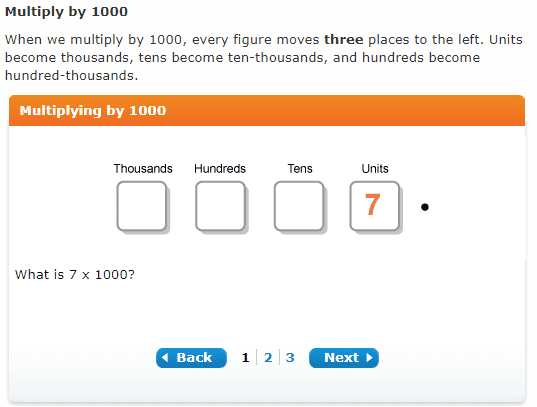http://www.bbc.co.uk/bitesize/ks3/maths/number/whole_numbers/revision/5/

What if we wanted to make enough lemonade to share between lots of people? What size container would we need? What if our recipe was for:

150 ml of freshly squeezed lemon juice

150 ml of syrup

700 ml of water? That adds up to

1,000 ml. That is the same as a litre.

1 ml is one-thousandth of a litre, so

To convert smaller units into bigger units, divide

to convert ml into L, we DIVIDE by 1,000.

1,000 ml ÷ 1,000 = 1L

(That is a real recipe by the way, and makes great lemonade.)

Click on the link below. Go straight to dividing by 1,000, and do parts 1, 2 and 3 coloured blue.

Remember: to convert smaller units into bigger units DIVIDE by 1,000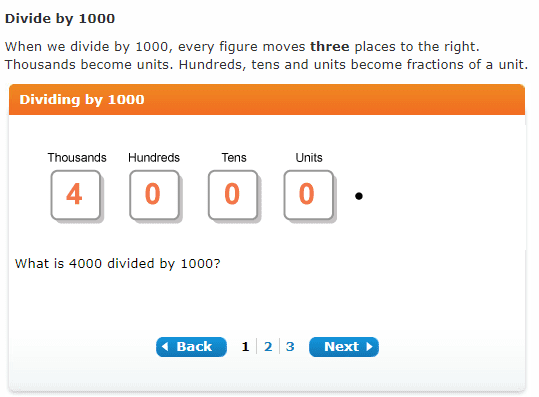http://www.bbc.co.uk/bitesize/ks3/maths/number/whole_numbers/revision/6/

Milli means one-thousand in Latin.

Kilo means one-thousand in Greek.

Here’s a clip.Weight/Mass

We need to be able to convert units of weight.

Sometimes we need stuff to be in big containers of a kilogram, (kg) to transport them from the shop to our cupboard. But then we need to use much less for our recipes. That is why we need smaller units called grams (g).

To convert between the bigger unit to the smaller units, we multiply

To convert between kg and g we MULTIPLY by 1, 000 because three are 1,000 grams in every kilogram.

1kg x 1,000 = 1,000g.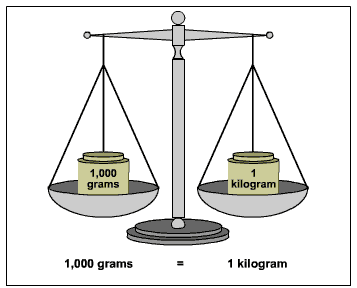What if we need to do it the other way around and convert grams into kilograms? One g is one-thousandth of a kg so

To convert smaller units into bigger units, we DIVIDE.

To convert g to kg we DIVIDE by 1,000.

1,000 g divided by 1,000 = 1kg.

Length

Length is measured in kilometers (or km) and metres (or m). To convert from bigger units to smaller units we MULTIPLY, so to convert from km to m we multiply by 1, 000.

We also split metres into centimetres, and centimetres into millimitres.

Centi means one-hundred, centimetres means one-hundredth of a metre.

We can split centimetres into even smaller units called millimetres. There are one-thousand millimetres in a metre.http://www.bbc.co.uk/bitesize/ks3/maths/number/whole_numbers/revision/5/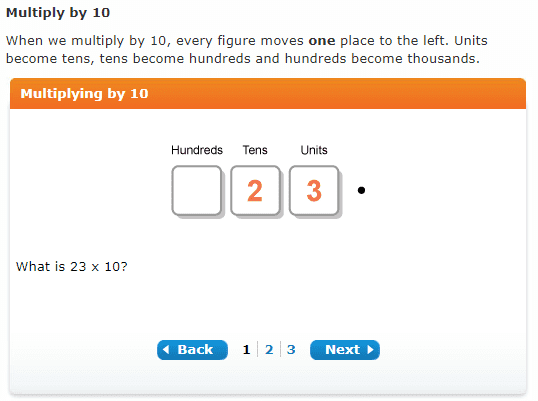http://www.bbc.co.uk/bitesize/ks3/maths/number/whole_numbers/revision/5/

If you were asked to convert cm into km, you would take it step-by-step: first convert cm into m, and the m into km.To convert from smaller units to bigger units, we DIVIDE.

More practice converting:http://flash.topmarks.co.uk/674

Remember the formulae:

To convert from bigger unit of measurement to smaller unit multiply:

1L into ml, multiply by 1, 000

Kg to g multiply by 1, 000

km to m multiply by 1, 000

To convert from smaller to bigger unit of measurement divide:

Ml into L, we divide by 1,000

G to kg divide by 1, 000

M to km, divide by 1,000

https://uk.ixl.com/math/year-5/compare-and-convert-metric-units-of-length

Practice Questions:

1. Convert 1kg to grams
2. Convert 2 kg into grams
3. Convert 1km into metres
4. Convert 2,000m into kms.
5. Convert 100cm into m
6. Convert 10 mm to cm
7. Convert 100 mm to com
8. Convert 1km into cm (take it a step at a time)
9. Convert 3,000 ml into L
10. Convert 1L into ml

Sometimes these conversions between units may involve decimal numbers. The same rules apply, but with decimals...

To convert from bigger unit of measurement to smaller unit multiply:

1.5L into ml, multiply by 1, 000

1.2 Kg to g multiply by 1, 000

2.5 km to m multiply by 1, 000

To convert from smaller to bigger unit of measurement divide:

1,500 Ml into L, we divide by 1,000

1,800 G to kg divide by 1, 000

1, 250 M to km, divide by 1,000

Practise dividing decimals.

Divide decimals of length by 1,000 while thinking of ml to L, g to kg, and m to km.

Divide decimals of length by 100 while thinking of converting centimetres to metres.

Divide decimals of length by 10 while thinking of converting between cm to m.

From smaller units to bigger unit, divide.http://www.bbc.co.uk/bitesize/ks3/maths/number/decimals/revision/5/Practice multiplying decimals by 1,000 while thinking of converting kg to g, L to ml and km to m.From bigger to smaller units, multiply.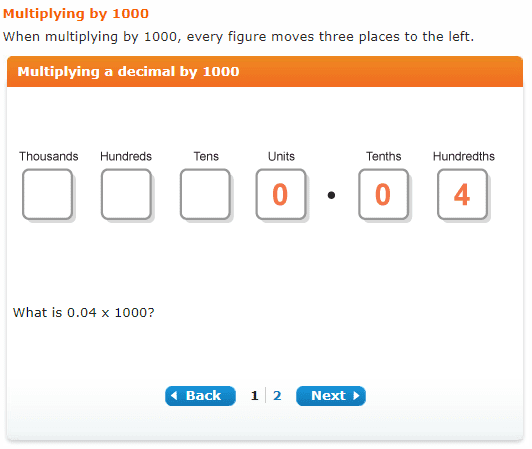http://www.bbc.co.uk/bitesize/ks3/maths/number/decimals/revision/4/

Multiply decimals by 100 while thinking of converting m to cmMultiply decimals by 10 while thinking of converting cm to mmFrom bigger unit to smaller unit, multiply.

Practise

1. Convert 2.5km into m
2. Convert 2.5 km into cm
3. Convert 1.7kg into g
4. Convert 0.5L into ml
5. Convert 15.65L into ml
6. Convert 750ml into L
7. Convert 500m into km
8. Convert 250g into kg
9. Convert 1500g into kg
10. Convert 10g into kg

If you need more practice on converting from l to ml, kg to g, km to m, the following link has more information and practice.

Non-Verbal Reasoning

Continued from last week:

Put it on full screen so you can see better:

With this type of question, you first need to focus on the two first shapes to spot the difference between one and the other. You will get confused if you don’t get this straight in your head first. After that, look at the third shape, and find the fourth one that changes in the same ways as the first one.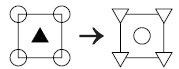Shape 1 and Shape 2. The circles on the corners change into triangles. The triangle changes into a circle, and changes shading from black to white. (Notice that the triangle on the inside of shape 1 has gone to the outer corners of shape 2, and the circle shape 2 may have come from the outer corners on shape 1. They have swapped.)

After that, look at the third shape.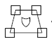Now find the shape ABCD or E that changes in the same ways.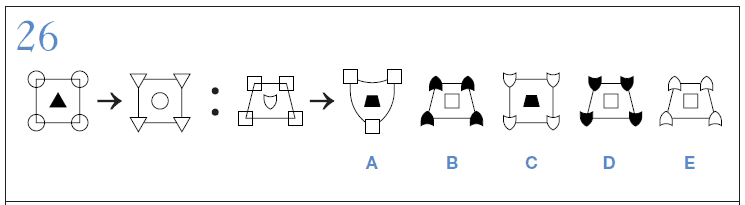Tip for 27.

Break each shape up into smaller parts. The first shape looks like dominos, with different shadings. The second shape also looks like dominos but they have moved position, and one of the dominos has split into two. The third shape is not made up of dominos, but triangular shapes similar to dominos. Look carefully at the shadings, and you will be able to work out the answer.Tip for 28. Outer lines, shapes and size feature here.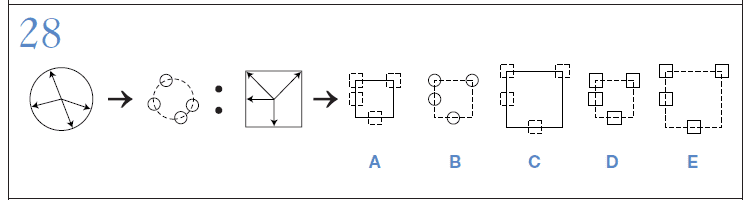Tip for 29. Look at left and right as well as the shading.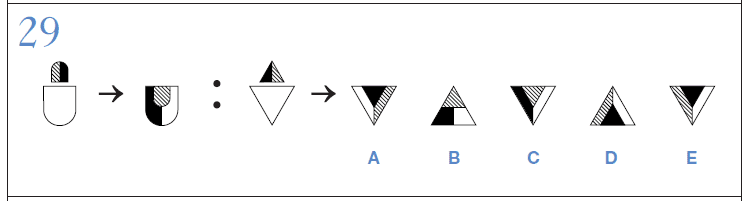This is a tricky shape, but take one bit at a time. You may want to focus on the arrowhead tip. It has tipped 180, and so has the larger hexagon shape and the inner shading.Verbal Reasoning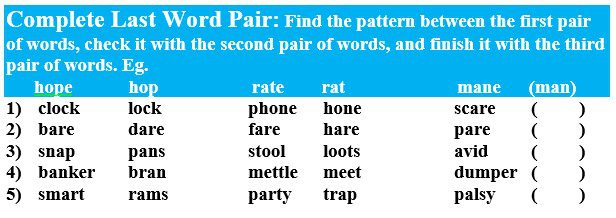English

Verbs are doing words or being words. Every sentence needs a verb. You can tell the verb because you can put the word ‘to’ in front of it and it makes sense.

Eg: to walk, to sit, to be.

Verb ‘to be’ is very commonly used in English. It is an irregular verb, which means we don’t say I be, you be, we beed, because it sound daft, right?

We say am, is, are, was, were, have been. Remember these little words because if they are in a sentence they are the verb.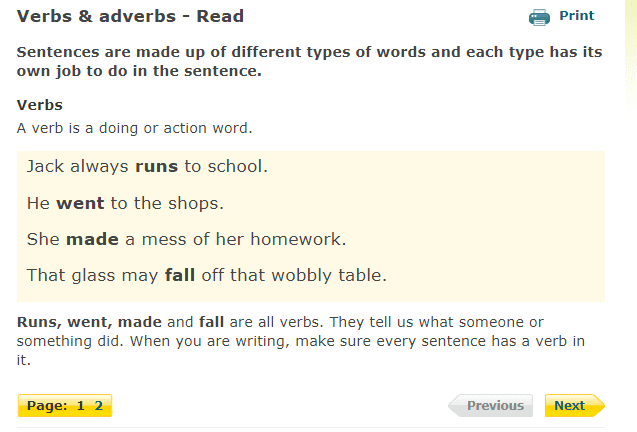Read over the irregular verbs listed below and make sure you know them. Write down the ones that surprised you, as this will help you remember them.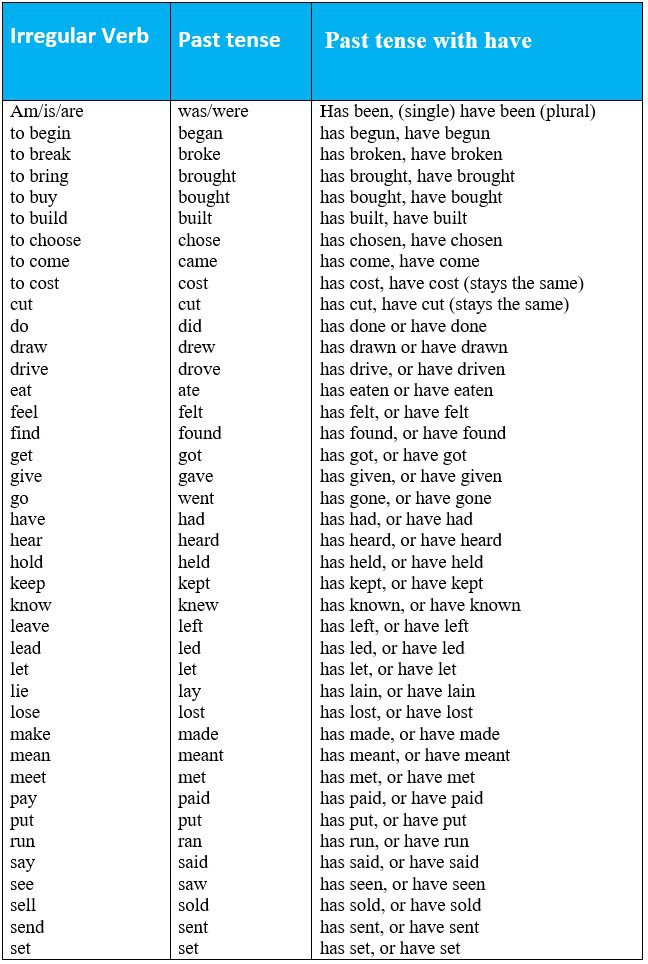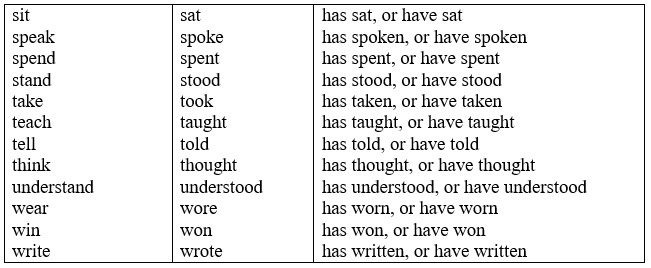ING Words

-ING Words need help to function as a verb.

They need help from the verb ‘to be.’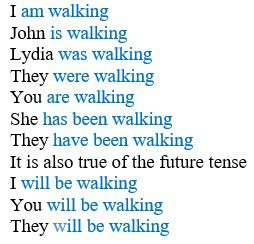Walking, does not function as a verb. Eg, ‘walking stick’. The stick is not walking. ‘Walking stick’ only describes the stick. Here the –ing word is a gerund, a verb that is half-adjective.

Walking is good for you. Here ‘walking’ is a gerund, a verb that is half-noun.

Adverbs: Ad – means towards, so adverb means ‘towards the verb.’ Adverbs describe HOW the action happened or WHEN the action happened and often end in –ly.

http://www.bbc.co.uk/bitesize/quiz/q25479810http://www.scootle.edu.au/ec/viewing/L6187/index.htmlVocabulary

Linking new words to words we already know helps to ‘hook’ them in our memory.

The abrupt eruption – think of volcanoes they explode suddenly. Abrupt means sudden.

Absurd - Rhymes with bird. It means ridiculous, or silly. Think of an absurd bird.

Picturing

Draw that absurd bird you imagined.

Abundant –means ‘lots of,’ so think of lots of buns

Here’s a list of the best 100 books ever. They will all be available in your local library, and some will be in your school library.

https://www.booktrust.org.uk/booklists/1/100-best-books-9-11/

Read anything, including comics on your favourite topic. It doesn’t have to be a book.